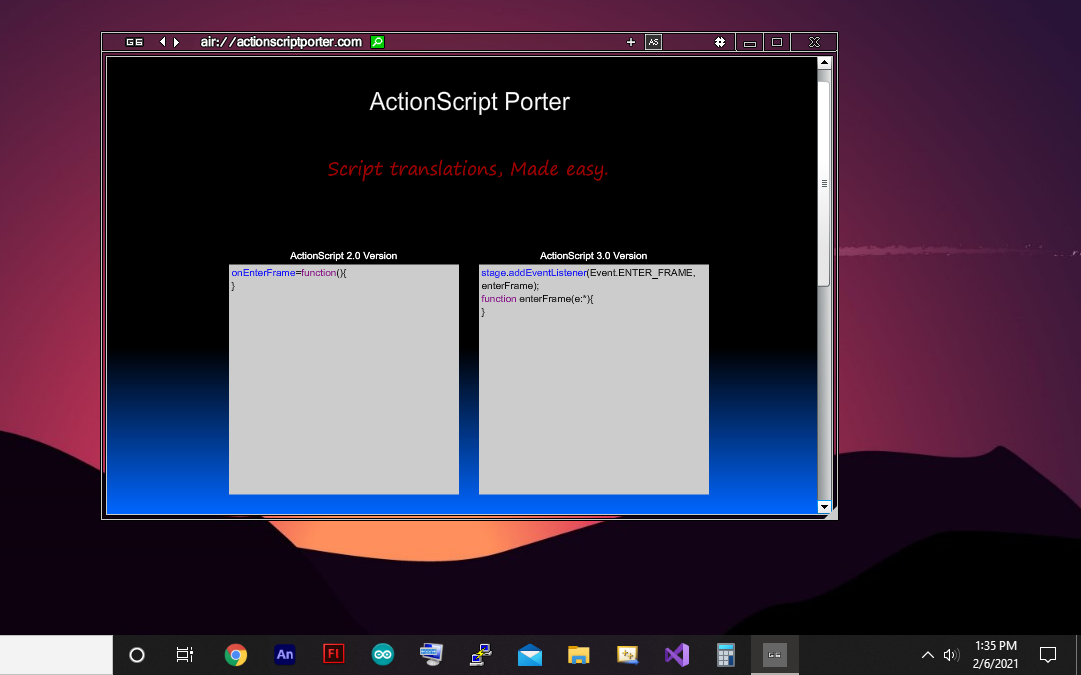A Flash Developer Resource Site

# Thread: any idea to play ASC2 SWF on android

1. ## any idea to play ASC2 SWF on android

hello all

i have actionscript 2 swf file

how to play it on android

thanks for helpReply With Quote

2. Yes. You can keep doing main timeline as2 if you are already comfortable with that language. I have a program I am writing for programmers like you called actionscript porter, where you paste your actionscript 2.0 and it outputs in actionscript 3.0 same thing, much like a english to spanish translator.

And for the android part I have this program I am designing for developers to present there 480x480 .swf in an android feed.

'Exec Post' is a social network built using adobe air where developers can share there latest capabilities in software through a single interactive static sized post (480x480 swf) that is ready to be viewed and tried by other developers and users with general feedback options to the developer.

version 0.1 - in progressReply With Quote

3. hello AS3.0

excuse me i dont understand you

can you explain step by step

thanks for helpReply With Quote

4. You will be able to present as2 on android when I finish my program and the as2 file needs to be 480 x 480 pxReply With Quote

5. this is the program that I will complete for your porting.Reply With Quote

6. I am waiting for this program and a video explanation

thanks for helpReply With Quote

7. Hello kofa, I am just letting you know that I am making progress in the as2 > as3 convertor, Here is a snippet of what the program is looking like:

PHP Code:
``` var instruction_set="" function processAS2(a){ var str="" instruction_set="" var last_syntax=null var quo_num_reformat=[]; for(var i=0;i<a.length;i++){ if(quo_num_reformat.length%2==0||quo_num_reformat.length==0){ if(a.text.substr(i,8)=="function"){ instruction_set+="function;" i+=8 } if(a.text.substr(i,2)=="if"){ instruction_set+="if;" i+=2 } if(a.text.substr(i,5)=="while"){ instruction_set+="while;" i+=5 } if(a.text.substr(i,3)=="for"){ instruction_set+="for;" i+=3 } if(a.text.substr(i,1)=="("){ instruction_set+="parenthesis_open;" i+=1 } if(a.text.substr(i,1)==")"){ instruction_set+="parenthesis_close;" i+=1 } if(a.text.substr(i,1)=="{"){ instruction_set+="curly_bracket_open;" i+=1 } if(a.text.substr(i,1)=="}"){ instruction_set+="curly_bracket_close;" i+=1 } }else{ str+=a.text.substr(i,1) } if(a.text.substr(i,1)=="\""){ quo_num_reformat.push(i); if(quo_num_reformat.length%2==0&&a.text.charAt(i+1)!=" "&&a.text.charAt(i+1)!=";"){ str+=";" } } } trace(instruction_set) quo_num_reformat=[] }  ```Reply With Quote

8. hello AS3.0

It's a great job, and because of that, can you edit swishmax and make it support Actionscript3

I imagine this would be the greatest

good luckReply With Quote

9. Yes. I worked on the convertors function called omitComments today which goes through the script to remove any script comments while allowing comment text in double primes and single primes so that when the reformatting processor occurs the whole script wont be read as a comment when it gets truncated:

input:
PHP Code:
``` var test = "//hey"//hey var lol="hi" //hey var test3 = "'//hey'" var test4 = '"//hey"'  ```
output:
PHP Code:
``` var test = "//hey" var lol="hi" var test3 = "'//hey'" var test4 = '"//hey"'  ```
snippet:
PHP Code:
``` var rebuild_string="" var mode=null; function omitComments(a){ var array = port_area.as2_field.text.split("\r")      rebuild_string="" quo_num=[] for(var j=0;j<array.length;j++){ for(var i=0;i<array[j].length;i++){ if(array[j].substr(i,1)=="\""&&quo_num.length==0){ quo_num.push(i); mode="\"" }else if(array[j].substr(i,1)=="'"&&quo_num.length==0){ quo_num.push(i); mode="'" }else if(mode=="'"&&array[j].substr(i,1)=="'"){ quo_num.push(i); }else if(mode=="\""&&array[j].substr(i,1)=="\""){ quo_num.push(i); } if(quo_num.length==0||quo_num.length%2==0){ if(array[j].substr(i,2)=="//"||i==array[j].length-1){ rebuild_string+=array[j].substr(0,i) if(array[j].charAt(i+1)!="/"){ rebuild_string+=array[j].charAt(i) } rebuild_string+="\r" quo_num=[] break; } //rebuild_string+=array[j].charAt(i) } } } trace(rebuild_string) }  ```Reply With Quote

10. very goodReply With Quote

####Posting Permissions

• You may not post new threads
• You may not post replies
• You may not post attachments
• You may not edit your posts
•

 » Home » Movies » Tutorials » Submissions » Board » Links » Reviews » Feedback » Gallery » Fonts » The Lounge » Sound Loops » Sound FX » About FK » Sitemap